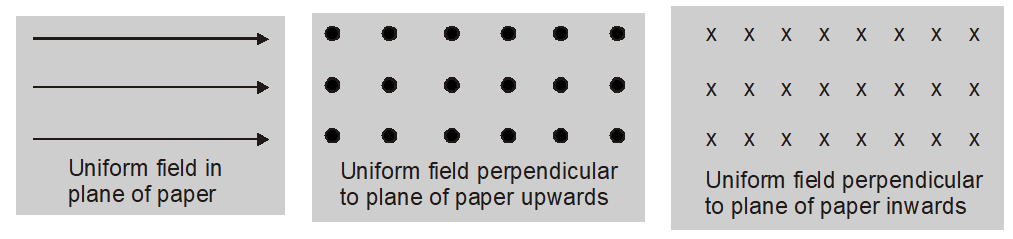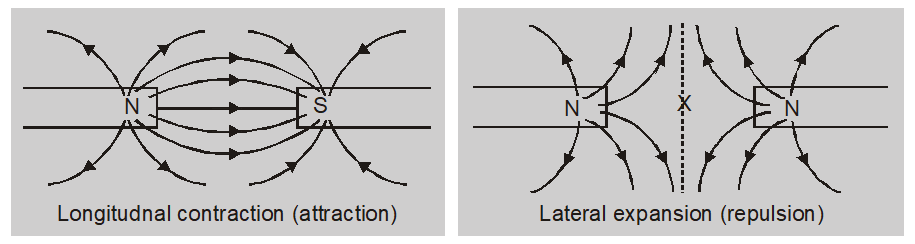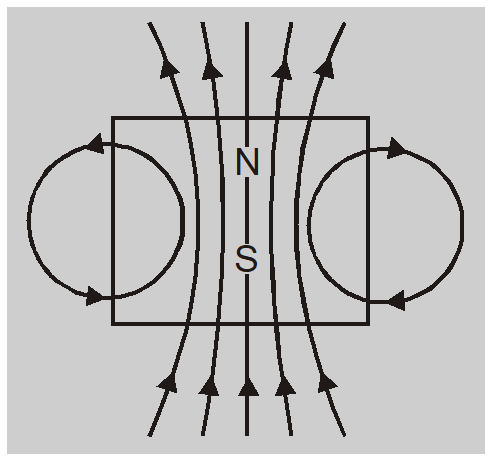Most Affordable JEE | NEET | 8,9,10 Preparation by Kota's Top IITian Doctor Faculties

# Magnetic Field | Properties of Magnetic Lines of Force Class 12, JEE## Magnetic Field

The space around a magnet (or a current-carrying conductor) in which its magnetic effect can be experienced. Magnetic Lines of Force can be defined as curved lines used to represent a magnetic field, drawn such that the number of lines relates to the magnetic field’s strength at a given point and the tangent of any curve at a particular point is along the direction of the magnetic force at that point. The Properties of Magnetic Lines of Force are also discussed.

## Magnetic Field:

Magnetic Field is defined as the space around a magnet (or a current-carrying conductor) in which its magnetic effect can be experienced.
1. The magnetic field in a region is said to be uniform if the magnitude of its strength and direction is the same at all points in that region.2. A magnetic field in a region is said to be uniform if the magnitude of its strength and direction is the same at all the points in that region.
3. The strength of the magnetic field is also known as magnetic induction or magnetic flux density.
4. The $\mathrm{SI}$ unit of the strength of the magnetic field is Tesla (T)1 Tesla = 1 newton ampere $^{-1}$ metre $^{-1}\left( NA ^{-1} m ^{-1}\right)=1$ Weber metre $^{-2}\left( Wb m ^{-2}\right)$
5. The CGS unit is Gauss (G)1 Gauss (G) $=10^{-4}$ Tesla (T)

### Properties of Magnetic Lines of Force:

The magnetic field lines are the graphical method of representation of the magnetic field. This was introduced by Michael Faraday.
1.  A line of force is an imaginary curve the tangent to which at a point gives the direction of the magnetic field at that point
2. The magnetic field line is the imaginary path along which an isolated north pole will tend to move if it is free to do so.
3. The magnetic lines of force are closed curves. They appear to converge or diverge at poles. outside the magnet, they run from north to south pole and inside from south to north.
4. The number of lines originating or terminating on a pole is proportional to its pole strength.Magnetic flux = number of magnetic lines of force = $\mu_{0} \times m$ Where $${\mu _0}$$ is a number of lines associated with a unit pole.
5. Magnetic lines of force do not intersect each other because if they do there will be two directions of the magnetic field which is not possible.
6. The magnetic lines of force may enter or come out of the surface at any angle.
7. The number of lines of force per unit area at a point gives the magnitude of the field at that point. The crowded lines show a strong field while distant lines represent a weak field.
8. The magnetic lines of force have a tendency to contract longitudinally like a stretched elastic string producing attraction between opposite poles.
9. The magnetic lines of force have a tendency to repel each other laterally resulting in repulsion between similar poles.10. The region of space with no magnetic field has no lines of force. At the neutral point where the resultant field is zero, there cannot be any line of force.
11. Magnetic lines of force exist inside every magnetized material.Important points :
1. Magnetic lines of force always form closed and continuous curves whereas the electric lines of force are discontinuous.
2. Each electric line of force starts from a positive charge and ends at a negative charge. Electric lines of force are discontinuous because no such lines exist inside a charged body.
3. In magnetism, as there are no monopolies, therefore, the magnetic field lines will be along with closed loops with no starting or ending. The magnetic lines of force would pass through the body of the magnet.
4. At very far-off points, the lines due to an electric dipole and a magnetic dipole appear identical.

About eSaral At eSaral we are offering a complete platform for IIT-JEE & NEET preparation. The main mission behind eSaral is to provide education to each and every student in India by eliminating the Geographic and Economic factors, as a nation’s progress and development depends on the availability of quality education to each and every one. With the blend of education & technology, eSaral team made the learning personalized & adaptive for everyone.